# Assignments

1. Bookstore
A university bookstore claims that 50% of its customers are satisfied with the service and prices.
a. If this claim is true, what is the probability that in a random sample of 600 customers less than 45% are satisfied?
b. If you would reject the bookstore’s claim if the probability computed in a. will be less than 0.05%, how many customers at least should have expressed satisfaction?

Solution.
a. We denote the (population) probability that is claimed by the bookstore as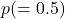and the probability computed by the sample as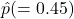. Then: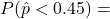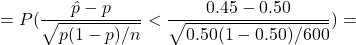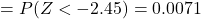b. In this case we have to computefrom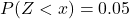. From table 3 in appendix B-8 we find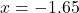. So, we have to solvefrom the equation: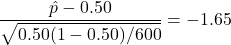and we get: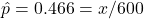and thus the number of customers expressing satisfaction should be at least 280.

0